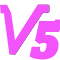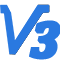barred galaxiesFourier decompositiongalaxy evolution

$\mathrm{A_{m}(r)=\frac {1}{\pi} \int_{0}^{2\pi}\sum_{(r, \theta)}cos(m\theta)d \theta},\ m=0,1,2,...$

$\mathrm{B_{m}(r)=\frac {1}{\pi} \int_{0}^{2\pi}\sum_{(r, \theta)}sin(m\theta)d \theta},\ m=0,1,2,...$

$\mathrm{A_m(R)=\sqrt{A_m^2+B_m^2}/A_0}$  （注：此处的 $\mathrm{A_m(R)}$ 与前面提到的$\mathrm{A_m(r)}$ 不同。

$\mathrm{A_m(R)}=\frac{|\sum_{j}m_{j}e^{mi\ \theta_j}|}{\sum_{j}m_j}$

$\mathrm{\Phi(R)=\frac{1}{m}arctan[\frac{\sum_{j}m_{j}sin(m\theta_j)}{\sum_{j}m_{j}cos(m\theta_j)}]}$

1. 强棒星系：$\mathrm{A_{2,max}}\ge 0.3$的盘星系，

2. 弱棒星系：$0.2\le\mathrm{A_{2,max}}< 0.3$的盘星系，

3. 非棒星系：不满足上述条件的剩下的所有盘星系。

3

1

{{notice.noticeContent}}
~~空空如也smith5个月14天前 IP:广东
922074

##### 200字以内，仅用于支线交流，主线讨论请采用回复功能。Yxiyns作者
5个月14天前 IP:福建
922078

1

##### 200字以内，仅用于支线交流，主线讨论请采用回复功能。Yxiyns作者
5个月13天前 IP:福建
922092

##### 200字以内，仅用于支线交流，主线讨论请采用回复功能。Yxiyns进士 机友 笔友

2

6

0
2017/04/10注册，1天16时前活动

IP归属地：未同步

{{errorInfo}}

##### 当前账号的附件下载数量限制如下：

{{f.startingTime}}点 - {{f.endTime}}点 {{f.fileCount}}

{{tip}}
{{reason.type}}

#### 空空如也

{{format('YYYY/MM/DD HH:mm:ss', toc)}}